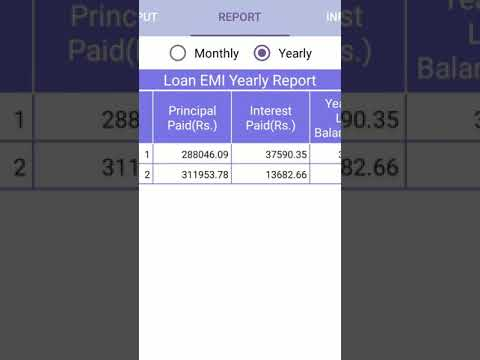# FinCal Plus - Financial CalculatorEveryoneFind all the free financial calculator in one place for India. Whether it is about future financial goals. This app can help you calculate all these in one click. Find out what will be the returns from all of your investments easily. This is the best online financial calculator app on the market. Financial Calculator app is a simple loan calculation tool that helps a user to quickly calculate EMI and view reports. Use the loan EMI calculator app to calculate your EMI, plan your mortgage repayments in an effective way.

You can calculate EMI for the home loan, car loan and other loan entities / online Mortgage Calculator EMI calculator section consist of basic / PRO / Advance EMI Calculator along with statistics. The banking calculation section consists of interest calculator on a financial instrument like Fixed Deposit / FD calculator, Recurring Deposit / RD calculator, Public provident fund / PPF calculator app for the people of India.

FEATURES:

* FREE
* Works on Smart Phones
* Exclusively for the people of India and NRIs
* Indian number format
* Displays the Maturity amount in reports
* Displays "Total Amount Deposited" and "Total Interest Earned"
* Displays Yearly and Monthly growth reports
* Contains in-built information about the Schemes details
* No Internet connection is required

Bank Calculators

# EMI Calculator.
# RD Calculator (recurring deposit calculator)
# Fixed Deposit Calculator (TDR - Interest Payout)
# Fixed Deposit Calculator (STDR - Cumulative)
# Loan Calculator.
# mortgage repayment calculator

Banking and Post Office Calculators

# Public Provident Fund Calculator(PPF Calculator)
# Kisan Vikas Patra Calculator. (KVP calculator)

1. EMI Calculator :-

EMI Calculator helps you to save your time. You need to just enter Loan Amount, Rate of Interest (per annum) and Loan Tenure period in years to calculate the EMI.

2. Fixed Deposit Calculator :-

Fixed Deposit (FD) Calculator helps you find out how much interest you can earn on Fixed deposite and the value of your investment on Maturity when interest is done on a Monthly, Quarterly basis.

3. Kisan Vikas Patra Calculator :-

Kisan Vikas Patra(KVP) Calculator helps you to calculate Maturity Date, Maturity Amount, Total Deposit by just entering your details like Deposit Amount, Rate of Interest (per annum) & Date of Account Opening.

4. Public Provident Fund Calculator :-

Public Provident Fund (PPF) Calculator helps the account holders to calculate interest on PPF Deposits and Maturity Amounts.

5. Recurring Deposit Calculator :-

Recurring Deposit(RD) Calculator help you to calculate Maturity Amount & you can easily visualize the calculation Graphically.

Collapse

Review Policy

Updated
July 22, 2019
Size
3.7M
Installs
50+
Current Version
2.3
Requires Android
4.1 and up
Content Rating
Everyone
Permissions
Offered By